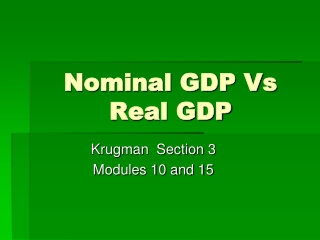DownloadDownload PresentationNominal GDP Vs Real GDP

# Nominal GDP Vs Real GDP

Télécharger la présentation## Nominal GDP Vs Real GDP

- - - - - - - - - - - - - - - - - - - - - - - - - - - E N D - - - - - - - - - - - - - - - - - - - - - - - - - - -
##### Presentation Transcript

1. Nominal GDP Vs Real GDP Krugman Section 3 Modules 10 and 15

2. GDP • Reminder: GDP is a figure including every item produced in the economy. • Money is the common denominator that allows us to add the total output.

3. GDPn • Is the market value of all final g & s produced in a year. • Calculated using current prices when the output was produced • Includes inflation • It is hard to compare market values from year to year when the value of the \$ itself changes (inflation or deflation) • To measure changes in the quantity of output, we need a “yardstick” that stays the same size.

4. GDPr • The value of the final g & s produced in a given year expressed in the prices of a base year • 2000 for our example

5. Traditional Method of Calculating GDPr • This economy produces apples & oranges • The base year is 2000. Since 2000 is the base year, real and nominal GDP are the same. • Only during the base year, will the GDPr and GDPn be the same

6. To find the GDPr in 2000, + the value of apples & oranges produced in 2000 using the table: Value of apples = 60 apples X \$.50 = \$30 Value of oranges = 80 oranges X \$.25 = \$20 • GDPr in 2000 = \$30 + \$20 = \$50

7. To calculate GDPr in 2006, + the value of apples and oranges using the prices of 2000 • Value of apples = 160 apples X \$.50 = \$80 • Value of oranges = 220 oranges X \$.25 = \$55 • GDPr in 2006 = \$80 + \$55 = \$135 • Nominal would be? • \$600

8. 2 purposes of estimating Real GDP • To compare the standard of living over time (based on quantity, not price) • To compare the standard of living among countries

9. Price Index • A measure of the price of a specified collection of g & s (market basket) in a given year as compared to the price of an identical collection of g & s in a reference year. • PI = price of market basket for a specific year X 100 price of same market basket in the base year • To find GDPr = GDPn / PI X 100

10. GDP Deflator • An average of current prices expressed as a percentage of base year prices. • Measures the price level • The average level of prices • GDP deflator = (GDPn / GDPr) X 100 • Example (\$100 / \$80) X 100 = GDP deflator • 1.25 X 100= 125 • Prices have gone up 25%

11. The Consumer Price Index(CPI) • Index the gov’t uses to measure inflation • Gov’t uses it to adjust SS benefits and income tax brackets • Reports 300 items in a market basket • Index or base year always = 100

12. Inflation • A rise in the general level of prices • Inflation rate = current CPI-Index CPI = rate (X 100)= % index CPI or Year2 – Year1 = rate (X 100) = % Year1 orYearAfter – YearBefore= rate (X 100) = % YearBefore